• Home• Patreon• Links• Books
Sturdy Lego Coupling:

# Converting Distance and Angle to X-Y Coordinates

Previously, I presented an overview of a sturdy Lego coupler. Before machining can begin, a layout must be drafted that matches the dimensions of the mounting holes in the Lego wheel. Attention students: If you are bored in math class wondering if there is any practical usage for what you’re learning, here’s a real-world example.Measurement of distance between cross axle hole and peg hole

The wheel has three peg holes around a center cross-axle hole. Each peg hole is exactly 8 mm (center-to-center) from the cross-axle hole. You can prove this to yourself by holding a Lego Technic beam in front of the wheel and rotating the wheel. Each of the outer holes lines up to exactly one Lego unit (LU) away from the center.

To capture what we know, let’s draw three holes that are 8 millimeters from the center and equidistant from each other.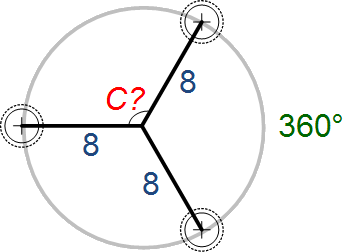Peg positions in Lego wheel hub

A circle is 360 degrees. There are three equal segments. So, the angle between segments is:

``` 360° ÷ 3 = 120° ```

To keep things simple, let’s ignore the third hole for the moment.Angle between hub peg holes

I need x-y coordinates for my milling machine digital readout. So, let’s add a 90 degree line from the center to the top hole to represent how far “up” the milling machine table needs to move.

``` 120° - 90° = 30° remaining ```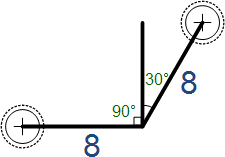Split angle at 90 degrees

How far to the right does the milling machine table need to move? Let’s connect the middle line to the right line with another 90 degree turn.I don’t care about the left hole anymore. It is easy to get to from the center, as the milling machine only needs to move 8 millimeters to the left. So, let’s drop that line segment and concentrate on the top-right hole.

That leaves a triangle with two known angles. The inside angles of a triangle always add up to 180 degrees. If we have already used up 90 degrees and 30 degrees, the missing angle must be:

``` 180° - 90° - 30° = 60° ```Angles of triangle for single peg hole

Beautiful.

I now have a triangle with all known angles and one known side. Better still, this is a special triangle called a 30-60-90 degree triangle. The relationships between the lengths of the sides of that type of triangle are mathematically well known.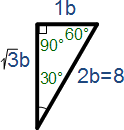Relationships of sides of 30 60 90 triangle for Lego hub

We know the length of one side is 8. Let’s solve for b.

``` 2b = 8 b = 8 / 2 b = 4 ```

Easy. Plug 4 in for b in the other formulas:

``` Length of top side = 1 * b Length of top side = 1 * 4 Length of top side = 4 ```
``` Length of left side = square root(3) * b Length of left side = square root(3) * 4 Length of left side = 1.73205 * 4 Length of left side = 6.9282 ```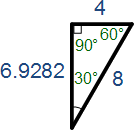Actual values for Lego peg holes triangle

My digital readout only displays two decimals places in metric. So, 6.9282 rounds up to 6.93. To drill the top hole, I need the milling table to go up 6.93 mm and over 4 mm. The bottom hole is in the same position, just down instead of up.

The finished coupler layout diagram looks like this.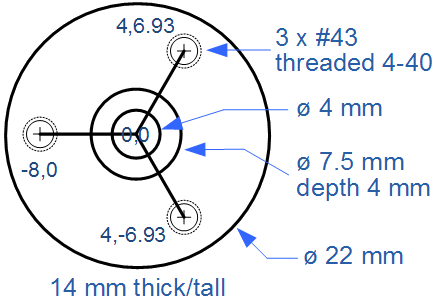Layout diagram for sturdy Lego coupler

From the center (0, 0) the three screw holes are located:

• Eight millimeters left (-8, 0)
• Four millimeters right and almost seven millimeters up (4, 6.93)
• Four millimeters right and almost seven millimeters down (4, -6.93)

The diagram shows that the three screw holes are drilled with a #43 drill designed for tapping 4-40 threads. The center hole is 4 millimeters in diameter (⌀) to match the gearmotor shaft. Another hole is drilled in the center to a depth of 4 millimeters and a diameter of 7.5 millimeters to accommodate the molded plastic where the cross axle attaches.

A total diameter of 22 millimeters was selected to fit within the inside of the smallest wheel hub. A thickness of 14 millimeters was chosen to hold the length of the motor shaft (10 millimeters) plus the molded cross-axle hole protrusion (4 mm).

We have everything we need to begin machining.